# Rectangle facts for kids

Kids Encyclopedia Facts
(Redirected from Oblong)

A rectangle is a shape with four sides and four corners. The corners are all right angles. It follows that the lengths of the pairs of sides opposite each other must be equal.

Tables, boxes, books, and papers look like rectangles.

A rectangle with all four sides equal in length is called a square.

## Formulas

If a rectangle has length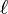$\ell$ and width w

• it has area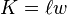$K = \ell w\,$,
• it has perimeter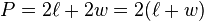$P = 2\ell + 2w = 2(\ell + w)\,$,
• each diagonal has length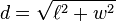$d=\sqrt{\ell^2 + w^2}$,
• and when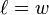$\ell = w\,$, the rectangle is a square.

## Images for kidsRectangle Facts for Kids. Kiddle Encyclopedia.